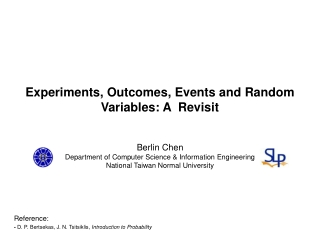DownloadDownload PresentationExperiments, Outcomes, Events and Random Variables: A Revisit

# Experiments, Outcomes, Events and Random Variables: A Revisit

Download Presentation## Experiments, Outcomes, Events and Random Variables: A Revisit

- - - - - - - - - - - - - - - - - - - - - - - - - - - E N D - - - - - - - - - - - - - - - - - - - - - - - - - - -
##### Presentation Transcript

1. Experiments, Outcomes, Events and Random Variables: A Revisit Berlin Chen Department of Computer Science & Information Engineering National Taiwan Normal University Reference: - D. P. Bertsekas, J. N. Tsitsiklis, Introduction to Probability

2. Experiments, Outcomes and Event • An experiment • Produces exactly one out of several possible outcomes • The set of all possible outcomes is called the sample space of the experiment, denoted by • A subset of the sample space (a collection of possible outcomes) is called an event • Examples of the experiment • A single toss of a coin (finite outcomes) • Three tosses of two dice (finite outcomes) • An infinite sequences of tosses of a coin (infinite outcomes) • Throwing a dart on a square (infinite outcomes), etc.

3. Probabilistic Models • A probabilistic model is a mathematical description of an uncertainty situation or an experiment • Elements of a probabilistic model • The sample space • The set of all possible outcomes of an experiment • The probability law • Assign to a set of possible outcomes (also called an event) a nonnegative number (called the probability of ) that encodes our knowledge or belief about the collective “likelihood” of the elements of

4. Three Probability Axioms • Nonnegativity • , for every event • Additivity • If and are two disjoint events, then the probability of their union satisfies • Normalization • The probability of the entire sample space is equal to 1, that is,

5. Random Variables • Given an experiment and the corresponding set of possible outcomes (the sample space), a random variable associates a particular number with each outcome • This number is referred to as the (numerical) value of the random variable • We can say a random variable is a real-valued function of the experimental outcome

6. Discrete/Continuous Random Variables (1/2) • A random variable is called discrete if its range (the set of values that it can take) is finite or at most countably infinite • A random variable is called continuous (not discrete) if its range (the set of values that it can take) is uncountably infinite • E.g., the experiment of choosing a point from the interval [−1, 1] • A random variable that associates the numerical value to the outcome is not discrete

7. Discrete/Continuous Random Variables (2/2) • A discrete random variable has an associated probability mass function(PMF), , which gives the probability of each numerical value that the random variable can take • A continuous random variable can be described in terms of a nonnegative function , called the probability density function(PDF) of, which satisfies for every subset B of the real line

8. Cumulative Distribution Functions (1/4) • The cumulative distribution function (CDF) of a random variable is denoted by and provides the probability • The CDF accumulates probability up to • The CDF provides a unified way to describe all kinds of random variables mathematically

9. Cumulative Distribution Functions (2/4) • The CDF is monotonically non-decreasing • The CDF tends to 0 as , and to 1 as • If is discrete, then is a piecewise constant function of

10. Cumulative Distribution Functions (3/4) • If is continuous, then is a continuous function of

11. Cumulative Distribution Functions (4/4) • If is discrete and takes integer values, the PMF and the CDF can be obtained from each other by summing or differencing • If is continuous, the PDF and the CDF can be obtained from each other by integration or differentiation • The second equality is valid for those for which the CDF has a derivative (e.g., the piecewise constant random variable)

12. Conditioning • Let and be two random variables associated with the same experiment • If and are discrete, the conditional PMF of is defined as ( where ) • If and are continuous, the conditional PDF of is defined as ( where )

13. Independence • Two random variables and are independent if • If two random variables and are independent (If and are discrete) (If and are continuous) (If and are discrete) (If and are continuous)

14. Expectation and Moments • The expectation of a random variable is defined by or • The n-thmoment of a random variable is the expected value of a random variable (or the random variable or • The 1st moment of a random variable is just its mean (If is discrete) (If is continuous) (If is discrete) (If is continuous)

15. Variance • The variance of a random variable is the expected value of a random variable • The standard derivation is another measure of dispersion, which is defined as (a square root of variance) • Easier to interpret, because it has the same units as

16. More Factors about Mean and Variance • Let be a random variable and let • If and are independent random variables and are functions of and ,respectively